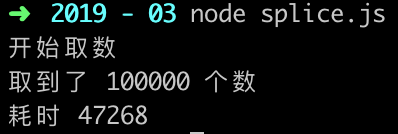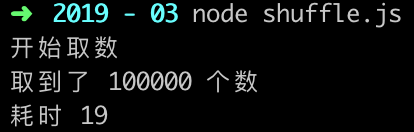# 前端面试(算法篇) - 数组乱序

##### 2019-03-12

`let randomIndex = arr[Math.floor(Math.random() * arr.length)];`

```function getRandomArrElement(arr, count) { let res = [] while (res.length < count) { // 生成随机 index
let randomIdx = (Math.random() * arr.length) >> 0; // splice 返回的是一个数组
res.push(arr.splice(randomIdx, 1)); } return res }```

```// 按位右移
(Math.random() * 100) >> 0

// Math.floor
Math.floor(Math.random() * 100) /* 这两种写法的结果是一样的，但位操作的效率更高 */```

sort() 会根据 compareFunction(a, b) 的返回值，来决定 a 和 b 的相对位置：

• 如果 compareFunction(a, b) 小于 0 ，那么 a 会被排列到 b 之前；
• 如果 compareFunction(a, b) 大于 0 ，那么 b 会被排列到 a 之前；
• 如果 compareFunction(a, b) 等于 0 ， a 和 b 的相对位置不变（不稳定！）

`function randomSort(a,b) { return .5 - Math.random(); } let arr = ['0', '1', '2', '3', '4', '5', '6', '7', '8', '9']; arr.sort(randomSort);`

`function shuffle(arr) { let len = arr.length; while (len) { let i = (Math.random() * len--) >> 0; // 交换位置 let temp = arr[len]; arr[len] = arr[i]; arr[i] = temp; } return arr; }`

`Array.prototype.shuffle = function() { let m = this.length, i; while (m) { i = (Math.random() * m--) >>> 0; [this[m], this[i]] = [this[i], this[m]] } return this; }``function getRandomArrElement(arr, count) { let shuffled = arr.slice(0), i = arr.length, min = i - count, temp, index; while (i > min) { index = Math.floor((i--) * Math.random()); temp = shuffled[index]; shuffled[index] = shuffled[i]; shuffled[i] = temp; } return shuffled.slice(min); }`然后是洗牌算法：《js随机数组，js随机洗牌算法》

《也谈前端面试常见问题之『数组乱序』》

《How to randomize (shuffle) a JavaScript array?》

### 最新文章

HashMap源码剖析

##### 2021-09-24

Mybatis中使用级联查询，一对多的查询

MySql分区、分表和分库

##### 2021-09-24

Batch Size对神经网络训练的影响

##### 2021-09-24

PC+PLC通过Modbus协议构建工控系统

##### 2021-09-24

element-ui的Tree树组件使用技巧

### 热门文章

##### 2019-08-27

【ZJOI2007】最大半联通子图

Mysql架构与内部模块-第二章

Nginx详解

##### 2019-08-18

Power BI-Power Pivot-客户最后下单时间（1）

##### 2019-08-10

Java——HashMap底层源码分析